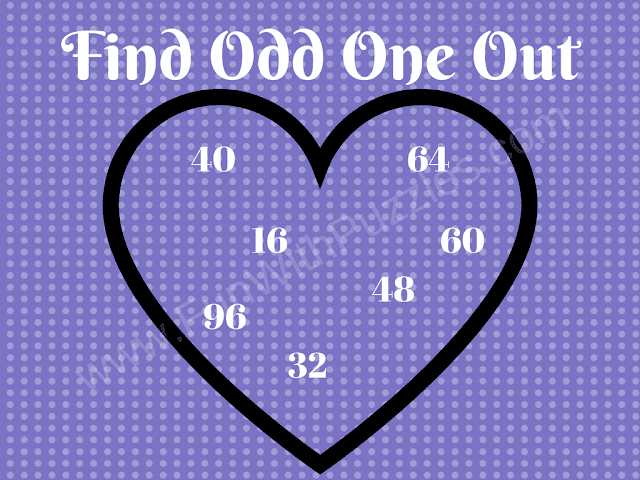Put your mathematical skills and logical thinking to the test with this Odd One Out Math Question. Find the number that doesn't belong in the heart-shaped puzzle. Are you up for the challenge?

Prepare to put your mathematical prowess and logical thinking to the test with this engaging Odd One Out Math Question. This mathematical challenge presents you with a heart-shaped puzzle containing a series of numbers. Your task is to identify the number that stands out from the rest.Odd One Out Math Question: A Mathematical Challenge

Solving this puzzle will require more than just basic math skills; it demands logical thinking and keen observation. As you examine the heart-shaped arrangement of numbers, pay close attention to the details, and identify the one number that doesn't quite fit the pattern.

Are you up for the challenge? Can you distinguish the Odd One Out among these numbers arranged in a heart shape? This brain teaser offers a fantastic opportunity to exercise your mathematical and logical thinking abilities while having fun with numbers.

The answer to this "Odd One Out Number Math Question", can be viewed by clicking the button. Please do give your best try before looking at the answer.

#### 1 comment:

Unknown said...

60, all the other number are divisible by 8